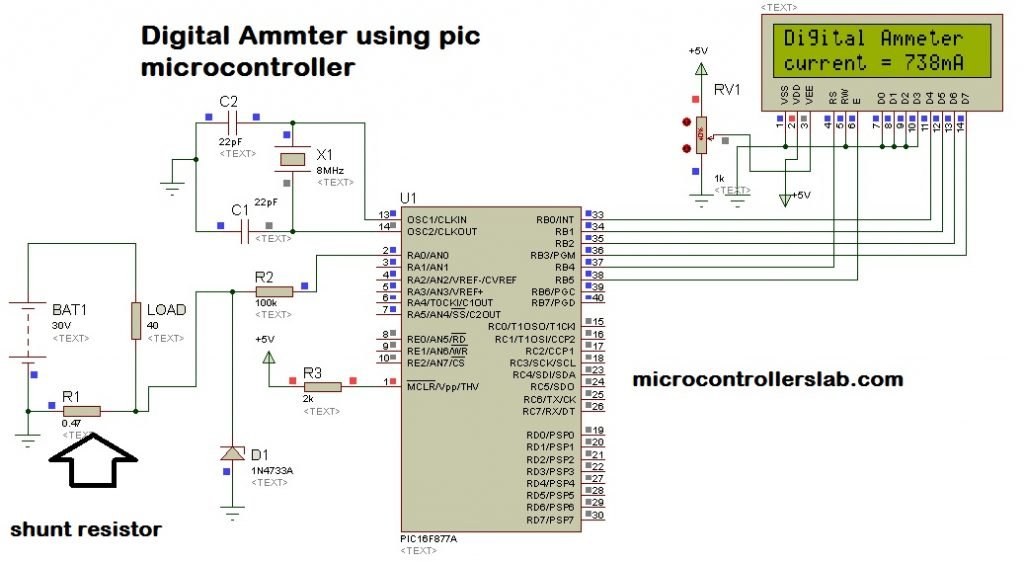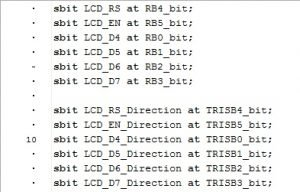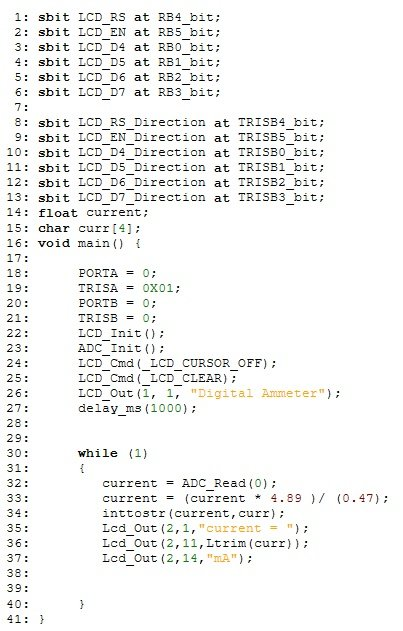# Digital Ammeter circuit using pic microcontroller

Digital Ammeter circuit using pic microcontroller is designed to measure dc current. In this project, you will learn how to measure dc current using pic microcontroller? how to convert current into voltage from using shunt resistor? how to design low resistance shunt resistor using simple wire?  How to display current value on LCD. Digital Ammeter using pic microcontroller project is designed to measure DC current. Digital AC Ammeter can also designed using pic microcontroller. Digital Ammeter circuit project has many application in electronics, electrical and power electronics projects. Current measurement is  also used in microcontroller based projects for over current protection in circuits. Other than this application, it can be used as digital Ammeter circuit for current measurement in electronics experiments.

### Circuit diagram of digital ammeter circuit using pic microcontroller:

Circuit diagram of digital Ammeter circuit using pic microcontroller is given below. In this circuit diagram, 30 volt dc source is applied to 40ohm load. Current passing through this load is measured with the help of shunt resistor and PIC16F877A microcontroller. Now the question is why we need shunt resistor to measure current?### Why we need shunt resistor?

Microcontrollers or any microcomputer system can not read current directly. Microcontrollers only sense voltage. Microcontrollers logic high and low is also based on voltage level. Therefore, microcontrollers do not sense current directly. That is why we need to current into voltage form. the Shunt resistor is used to convert current into voltage form. When current passes through shunt resistor, voltage appears across shunt resistor. This voltage can be easily measured with the help of analog to digital converter channel of PIC16F877A microcontroller. This measured voltage value can be converted back into current in programming using ohm’s law formula:

V = IR;

Shunt resistor value is known and voltage is measured with pic microcontroller. So current can be easily calculated by using above Ohm’s law formula.

I = V/R

In this digital ammeter circuit project, 0.47 ohm shunt resistor is used.  Now you must be wondering how to design this low resistance value resistor? The simplest solution to this problem is to take a piece of wire and makes it few turn. After making few turns, measure its resistance with the help of ohm meter. You can easily design .47 ohm resistor with the help of simple wire. It is a hit and trail method. Keep trying until you successfully get a value close to .47 ohm. Other option is to used hall effect current sensor which converts current into voltage directly. Magnitude of voltage depends on magnitude of current.

100K ohm resistor at the input of analog to digital converter is used to protect microcontroller from high current. because current always follow a low resistance path. If you don’t use any resistor  there, current will start to flow towards microcontroller instead of shunt resistor. It will damage microcontroller permanently.1N4733A zener diode is used to protect microcontroller from over voltage appearing across it. Because voltage more than 5 volt may damage microcontroller permanently. LCD is interfaced with PIC16F877A microcontroller to display current value. If you don’t know how to interface LCD with PIC16F877A microcontroller, check following article first:

### Program for digital ammeter circuit using pic microcontroller

Now lets discuss how to write code for digital ammeter using pic microcontroller? program for digital Ammeter is written using Mikro C pro compiler for pic. It is very easy to use to compiler for pic microcontroller. It have large set of libraries which makes microchip microcontroller very easy to use.

Following programming instructions defines that LCD is connected with PORTB of PIC16F877A microcontroller. PORTB is used to send data to LCD. These are simple lines like an english sentences tells microcontroller that LCD is connected to PORTB pins and these lines also specified the pin numbers as shown in figure below:Complete code of digital Ammeter circuit using pic microcontroller is given below. In this code TRISA is used to define port A as input because voltage is measured with pin number AN0 of port A. TRISB instructions defines PORTB as an output PORT. It is used to send data to LCD.  LCD_Init() funtion initialize LCD libraries and ADC_Init() initialized ADC libraries functions. “current = ADC_Read(0); “statement used to read voltage across shunt resistor and “current = (current * 4.89 )/ (0.47); ” statement converts voltage back into current according to ohms law formula. Rest all the lines in below given code is self explanatory, but if you still need any help. You are welcome to comment on this post.[sociallocker][/sociallocker]This is all about digital ammeter circuit using pic microcontroller project, circuit diagram and coding. If you feel any issue while designing your digital ammeter circuit, feel free to comment on this post. You may also check following articles:

### 21 thoughts on “Digital Ammeter circuit using pic microcontroller”

1. kindly tell me about why you multiply 4.89 to the voltage???

2. Hello,

Can you tell me why you are multiplying current with 4.89 in the expression “current=(current*4.89)/0.47” ? From where you got the value 4.89. And what will be the maximum current it can measure?

Thank you.

• It is reslution factor it is used to convert digital read value back into voltage form

3. The 0.47 resistance must have a specific watt?
Thanks for sharing !

4. What is the maximum current can be measured by this ammeter

5. What is the current range input

6. can measure high current?

• yes But it depends on wattage rating of shunt resistor. For example if you want to measure 10 Ampere current and You have used 0.1 Ohm shunt resistor. Then power rating of shunt resistor should be equal to P = I^2 X R = 10^2 X 0.1 = 10W. But if you can make shunt of value in the order of 0.001 ohm it will reduce losses

7. how wattage need to resistor shunt?

• simple ohm law V=IR use I = V /R Because you know V and R

9. aoa. i am confusing with ur breifing what u told in video to calculate current using cuurent transformer remember we are talking about dc and as we know in dc any transformer wont work,it will only wo when we are measuring ac currents, plz clarify this one.
thanks

10. I can’t get a 0.47ohm shunt resistor, but i have a 47 ohm resistor. Can i make this work?? Do I have to alter the code too???
Working on a similar project and I’d appreciate your speedy reply. Thanks

• U can’t 47 ohm is too bigger & the drop…makes hefty power loss

11. Can you send the C code for me?

My e-mail adress is [email protected]

Thanks a lot,

Cláudio

12. could u send the hex code for ammeter displays.

13. i need this function alone inttostr(current,curr) for micro controller ? can you share those function alone

14. can you send me the original C codes and also the hex code for ammeter display?
this is my email [email protected]
thanks a lot!